Economics
Volume 4, Issue 6, December 2015, Pages: 118-124

Emerging Relationships Between Macroeconomic Indicators and Real Economic Activities in Ghanaian Economy

Nana Kena Frempong, Peter Amoako-Yirenkyi, Akoto Yaw Omari-Sasu, Derrick Asamoah Owusu

Department of Mathematics, College of Science, Kwame Nkrumah University of Science and Technology, Kumasi, Ghana(N. K. Frempong)

Nana Kena Frempong, Peter Amoako-Yirenkyi, Akoto Yaw Omari-Sasu, Derrick Asamoah Owusu. Emerging Relationships Between Macroeconomic Indicators and Real Economic Activities in Ghanaian Economy. Economics. Vol. 4, No. 6, 2015, pp. 118-124. doi: 10.11648/j.eco.20150406.14

Abstract: The aim of this study is to empirically estimate the sensitivity of inflation and interest rate together with some key macroeconomic variables on real economic activity index. Secondly, to investigate the direction of causality between inflation and interest rate, interest rate and real economic activity index, as well as between inflation and real economic activity index. Thirdly, to investigate how the real economic activity reacts to shocks from inflation and interest rate and other key macroeconomic variables. A deterministic time series regression model, vector autoregressive model, cointegration method and Granger Causality Analysis were implored in the analysis. The results show that there exists a unique long-run relationship between real economic activity and the other variables. Accordingly, inflation is identified as important determinant of real economic activity growth in Ghana. Conversely, real economic activity was found not to significantly determine inflation and interest rate within the period under investigation. The relationship between inflation and interest rate is, however, significant determined positive in either direction. This means that the link between inflation, interest rate and economic activity is not immediately automatic.

Keywords: Macroeconomic, Vector Autoregressive, Cointegration, Granger Causality

1. Introduction

Macroeconomic indicators such as inflation and interest rates have been the leading actor in economic growth for most developing and industrialized nations in the last decade. One of the most fundamental objectives of macroeconomic policies in Ghana is to sustain high economic growth together with low inflation and low interest rates. There exists a considerable debate regarding the existence and nature of inflation and economic growth relationship. A study in south Asian countries shows a long-run positive relationship between GDP growth rate and inflation . A consensus, nonetheless exists, thus suggesting that macroeconomic stability, which is rooted in the spirit of low inflation, is positively related to economic growth. One of the macroeconomic growth factors is nominal interest rate and its fluctuating nature is closely related to inflation rate. Its vacillating trends also impact on economic boom or gross domestic product (GDP) and extending to influence economic growth rate . Thus, inflation has been touted as the main predictor of interest rates and consequently affecting real economic activities. Ideally, every economy would wish to achieve zero interest rate. A study in neighboring country shows that the behaviour of interest rate is important for economic growth in view of the relationships between interest rates and investment and investment and growth . Its direct mechanism is that, investment removes limitations of growth resulting from system oldness and its inability to achieve potential ability and new capital accumulation and technical progress allow reaching desirable capital volume. In theoretical models especially life cycle model, both roles are emphasized. In important experimental fields, actual interest rate is regarded as investment factor. It seems that demand for investment is not satisfactory in low interest rate.

An economic challenge that Ghana has experienced since independence is price determination and inflation on goods and services. Shortly after independence (i.e., 1957 – 1962), there was relative stability in the determination of prices as inflation vacillated around a single digit. Contrastingly, the years 1970s and 1980s recorded unprecedented macroeconomic instability and very high inflation episodes. Indeed, inflation overstepped 100 percent on four occasions between July 1977 and March 1983. Consequent upon this hapless achievement, Ghana embarked on a stabilization policy, dubbed the Economic Recovery Programme (ERP). This policy nonetheless appeared to have done a little in resolving the persistently high and vacillating inflation. Until recently, the country had not achieved single-digit inflation since the period promptly after independence and had always recorded annual average inflation rates of about 25% in more than half of those years. the recent single-digit achievement in inflation, though significant, seems to disconnect theoretically with some key macroeconomic variables like interest rates and exchange rates, money supply and price of crude oil.  studied the Granger Causality Analysis on Ghana’s Macro-Economic

Performance and Oil Price Fluctuations. They observed that a unidirectional causality runs from oil price fluctuations to output and economic growth in Ghana. In some other cases, the macroeconomic variables performance and stock market index have a long-run relationships (see ).

The problem we seek to solve is how does inflation and interest rate affect economic activity in Ghana.

The aim of this paper is threefold. Firstly, empirically estimate the sensitivity of inflation and interest rate together with some key macroeconomic variables on real economic activity index. Secondly, to investigate the direction of causality between inflation and interest rate, interest rate and real economic activity index, as well as between inflation and real economic activity index. Thirdly, to investigate how the real economic activity reacts to shocks from inflation and interest rate and other key macroeconomic variables. In particular we analyze and test the trends in inflation, interest rate and real economic activity in Ghana over the period November 1990 through to December 2011, in order to detect if inflation and interest rate are driven by a unique source of randomness, to detect the existence of cointegration.

The paper is organized as follows: Section 2 describes the data set given by monthly series over the period 1990 to 2011 and examines the transformation of each time series. In Sections 3 current state of the art methodologies are used to analyze the short- and long-run relationships, as well as a rolling correlation and cointegration approach. Section 4 discusses the results of the analysis and section 5 draws some preliminary conclusions. The statistical package Stata version 12.0 was used for the analysis.

2. Data

Monthly series on real economic activity index, inflation, interest rate and exchange rate span from the period November 1990 through to December 2011 was explored. Since GDP figures are unavailable on a monthly basis, we used a composite index for economic activity (CIEA) from the central Bank of Ghana as a proxy variable for the real economic activity. Nominal exchange rate is the nominal monthly average Ghana Cedi (GH¢)1 to US dollar (US\$) rate. Inflation is proxied by the average monthly inflation, while 91-day Treasury bill rate is used as a proxy for interest rates. We use M2+ (broad money supply including foreign currency deposits) as a proxy for money supply while external supply shocks are proxied using monthly crude oil prices. All data used in the analysis were obtained from different sources namely, Bank of Ghana, IMF, International Financial Statistics, Government Finance Statistics and the World Bank Development Indicators. Other augmenting sources include published articles and journals, working papers, textbooks and relevant internet resources. We log transform each of the time series data, the dynamics of the three main series are presented in figure 1 below: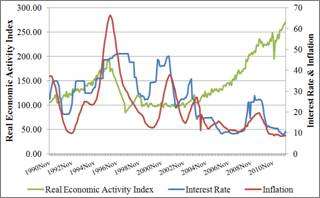Fig. 1. Time series evolution of real economic activity index, interest rate and inflation, 1990-2011.

A preliminary analysis is going to be performed on the stationarity of the three time series. In line with most of the recent literature, we transform the original series to natural logs. First we test the order of integration of a time series using the Augmented Dickey-Fuller (ADF) type regression.

3. Methodology

3.1. Model Specification

To study the empirical results of the relationship between inflation, interest rates and real economic activity index, we specify an augmented model for Ghana based on the theoretical and empirical literature. Specifically, following the neoclassical model (, , and ) specifications, the present study specify a model of the form: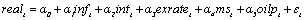(1)

where realt is a measure of economic activity index at time t ; inft is the rate of inflation at time t; exratet is the exchange rate at time t; intt is the interest rate at time t; mst is a measure of money supply (which captures the effect of financial deepening) at time t; oilpt is oil prices at time t to proxy external supply shocks which is also treated as an exogenous variable since Ghana until recently that it discovered oil in commercial quantities was a small oil importing country and hence cannot influence the world market price of oil; and εt is the usual white noise error term. We log transform the time series in equation (1) in order to interpret the coefficients as partial elasticity’s. Thus, the estimable deterministic long-run model in (1) is specified as follows: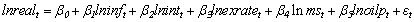(2)

The specific estimation technique used in this paper is cointegration. This statistical concept introduced by  has received wide in the literature. In this concept, two variables are cointegrated when a linear combination of the two is stationary, even though each variable is non-stationary. In order to avoid the problem of non-stationarity we make use of first (or higher) differenced data. Such differencing, however, may result in a loss of low frequency information or long-run characteristics in the series. However,  argued that, if there is an equilibrium relationship between such variables, then for this relationship to have any meaning, a linear combination of these variables the disequilibrium error should fluctuate about zero i.e. should be stationary. A two variable cointegration test requires that the variables be integrated of order one. A number of alternative tests are available for testing whether a series is stationary or not, the Augmented Dickey-Fuller (ADF), developed by , as well as the Phillips Perron (PP) test developed by . The PP tests are based on the following ADF regression, and the critical values are the same as those used for the ADF tests: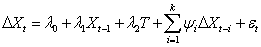(3)

whereis the difference operator, X is the natural logarithm of the series, T is a trend variable,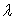and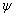are the parameters to be estimated and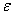is the error term. The PP unit root test corrects the serial correlation and autoregressive heteroscedasticity of the error terms. This aims at providing unit root tests results that are robust to serial correlation and time dependent heteroscedasticity of errors. In both the PP and ADF unit root tests the null hypothesis is that the series is non-stationary and this is either accepted or rejected by examination of the t-ratio of the lagged term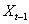compared with the tabulated values. If the t-ratio is less than the critical value the null hypothesis of a unit root (i.e. the series is non-stationary) is accepted. If so the first difference of the series is evaluated by equation (3) and if the null hypothesis is rejected the series is considered stationary and the assumption is that the series is integrated of order one I(1). Critical values for this t-statistic are given by .

3.2. Multivariate Cointegration Model

The Vector Autoregression (VAR) approach is employed in this paper. This model avoids the need to provide a dynamic theory specifying the relationships among the jointly determined variables. Moreover, it can handle endogenous variables on both sides of the equation as well as a mix of I(1) and I(0) variables in one system. In a VAR system, each variable is regressed on its own lags plus the lags of the other variables. The appropriate lag length (p), which should be specified long enough for the residuals not to be serially correlated, can be determined using standard model selection criteria such as the Akaike information criterion (AIC), Schwarz information criterion (SIC), Hannan-Quinn information criterion (HQ) and the Final prediction error (FPE) information criteria. Assume Yt is a vector of k jointly determined endogenous variables and Xt is a vector of m exogenous variables. A pth order VAR model of the inter-related time series, VAR (p), can be written as: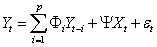(4)

where Φi and Ψ are matrices of coefficients to be estimated, andis a vector of independent and identically distributed disturbances. If the endogenous variables are each I(1) we can write the VAR(p) model in (4) as a vector error correction model (VECM):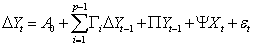(5)

where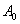is avector of constants,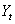is anvector of I(1) variable, p is the number of lagsand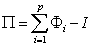, Δ is a difference operator, I is a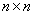vector of identity matrix and εt isvector of independently and identically distributed error terms. Granger’s Representation Theorem asserts that if the coefficient matrix Π has reduced rank (i.e., rank (Π) =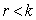) then there exist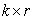matrices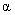andeach with rank r such that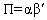and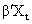is I(0). The rank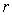is the number of cointegrating relations and each column ofis the cointegrating vector (CV). The Johansen maximum likelihood estimation procedure (, ) can be used to estimate the two matricesandand test for the number of distinct CVs. Restrictions on the elements ofhelp to determine which variables are relevant in the long-run relations; economic theory may have to be invoked to decide on the restrictions to impose on each CV . The elements ofare known as the adjustment parameters in the VECM. The hypothesis that Π has a reduced rank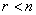is tested using the trace and the maximum eigenvalues test statistics. Restrictions on the adjustment parameters help to determine which variables are weakly exogenous. The impulse response functions (IRFs) generated from the VAR model trace out the time paths of the effect of a shock in a nominated variable on each of the other variables in the system. From them we can determine the extent to which an exogenous shock causes short-run and long-run changes in the respective variables.

The vector error-correction model (VECM) with a lag order p is specifically modeled as follows: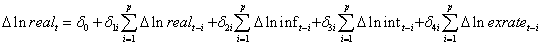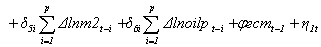(6)

where all variables are as previously defined,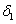,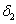,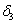,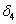,, and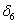represent short-run elasticities,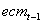is the error correction term with its coefficient,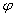denoting the speed of adjustment to long-run equilibrium after a shock to the system.

3.3. Granger Causality Analysis

The final stage of the estimation involves conducting Granger causality tests to find the direction of causality and possible feedback between real economic activity, inflation, interest rates and the other determinants of real economic activity. By Granger’s representation theorem, if variables are cointegrated, there must be causality in at least one direction and the long run relationship is free of spurious correlations. Granger causality tests whether lagged values of one variable predict changes in another, or whether one variable in the system explains the time path of the other variables. Hence, a variable x is said to Granger cause another variable y (x y) if past values of x can predict present values of y.  posits two cardinal principles namely the cause precedes the effect and; ‘the causal series contains special information about the series being caused that is not available in the other available series’ . Similarly, there is an instantaneous causality from x to y (xy) if present and past values of x predict present value of y. If causality is in one direction e.g. from x to y, we have uni-directional causality while if x Granger causes y and y Granger causes x, we have bi-directional or feedback causality (yx). There are two commonly used causality tests: one due to  and others. The former is, however, more widely used in applied econometrics, partly because of its simplicity and also because it is less costly in terms of degrees of freedom. The Granger-causality test is based on the assumption of stationary variables. Thus, if the variables are non-stationary then, Granger-causality tests are applied on the first differences of the variables that have unit root. The test for Granger causality is performed by estimating equations of the following form:(7)

The F-test is applied to test the null hypothesis of Granger-non-causality against the alternative of Granger-causality. If the F-statistic is significant at any of the conventional levels, the null hypothesis that ‘Y does not Granger-cause X’ is rejected, otherwise it is accepted.

4. Results

4.1. Descriptive Statistics and Pair-Wise Correlation of the Variables

From fig 1 under section 2, we observe a general trend between interest rate series and real economic activity index series in the sense that, periods of high interest rates shows periods of relatively low economic activities and vice versa. The fig 1 also depicts inflation series appears to have sustained fluctuating trends, though generally downwards, whiles the real activity fluctuates frequently upwards. Table 1 below presents the descriptive statistics for the macroeconomic variables.

Table 1. Summary statistics of the logged series.

 Series Obs Mean Std. Dev. Min Max lnreal 254 4.912 0.293 4.437 5.603 lnint 254 3.180 0.509 2.213 3.870 lninf 254 2.945 0.506 2.127 4.196 lnm2 254 6.649 1.876 3.346 9.818 lnoilp 254 3.449 0.659 2.343 4.887 lnexrate 254 -0.982 1.197 -3.370 0.460

All variables exhibit a positive mean rate except for exchange rate, which shows a decline on the average by 37% within the period of observation. Table 2.0 also presents the pairwise unconditional correlations between the various series. This is to identify variables that could potentially cause multicollinearity in the model. The results indicate that real economic activity is negatively correlated with both inflation and interest rates. However, the correlation is somewhat weak. Inflation and interest rate are positively correlated with the magnitude indicating a fairly high correlation. It is, however, important to emphasize that, correlation does not necessarily imply causation. Thus, latter sections would seek to establish a causal linkage amongst these variables of interest.

Table 2. Sample Correlation Matrix.

 series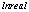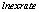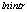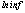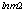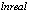1.0000 0.3354 -0.4469 -0.2716 0.5108 0.62921.0000 -0.5241 -0.4182 0.9678 0.7624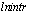1.0000 0.7736 -0.6512 -0.7805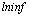1.0000 -0.5244 -0.6184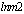1.0000 0.8632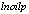1.0000

The relationship to be investigated between real economic activity and macroeconomic variables specified in equation (2) was fitted and the following Ordinary Least Squares estimates were obtained as shown in table 2 below: We observe from table 3, that the significant predictors for real economic activity are exchange rate, interest rate and inflation. This model explains about 77% of the total variations in the real economic activity index series. It must be emphasized, however, that the result are not to be given absolute relevance since the variables at their levels are potentially non-stationary. These preliminary results identified as to which variables are appropriate to be included in the final analysis.

Table 3. Parameter estimates for specified model in (2).

 Coefficient Estimate Std.error Constant 1.157** (0.521) time 0.014*** (0.003)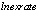-0.696*** (0.039)0.141*** (0.035)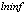0.212*** (0.030)0.007 (0.127)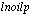0.054 (0.038) Adj R2 0.773

To establish a long run relationship amongst the variables, and to avert false regression, the order of integration of each of the variables is inspected using the Augmented Dickey-Fuller (ADF) unit root tests. The results of the unit root test are reported in Table 4 below.

Table 4. Unit root test results for the logged series.

 Level First Difference Series Constant Constant Series Constant Constant No Trend Trend No Trend Trend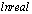-0.102 -0.829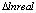-3.389** -3.647**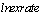-2.778* -1.435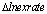-3.161** -3.838**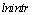-1.161 -2.861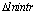-4.464*** -4.590***-1.195 -2.402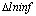-4.398*** -4.512***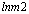-1.553 -3.284*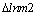-2.914** -3.159*0.072 -2.424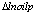-5.114*** -5.232*** The null hypothesis is that the series is non-stationary, or contains a unit root. The rejection of the null hypothesis based on the MacKinnon critical values. *, ** and *** indicate the rejection of the null hypothesis at 10%, 5% and 1% significance level, respectively.

We run the test without any exogenous variable, with a constant and a constant plus a linear time trend as exogenous variables in equation (3). The results indicates that, each of the series is integrated of order one (i.e, I(1)), and thus contain unit root. It is relevant to stress that, achieving stationarity is a precondition for the estimating our VAR model in equation (4) and consequently cointegration analysis.

4.2. Cointegration Test Results

We examine the potential influences of important exogenous factors. Consequently, we incorporate in the model oil price to capture the influence of external shocks or supply shocks.2 Thus, a general-to-specific modeling approach  led to the selection of a VAR model of order 2 (that is, VAR(2)).3 Table 5 indicates that, the optimal lag to be used in the VAR is 2 according to the AIC criteria. The motivation is not to interpret the coefficients in the VAR but to be used as a basis to estimate the cointegrating relationship between real economic activity and its determinants. As a consequence, we performed a battery of diagnostics on the estimated VAR model of serial correlation, stability and normality of the residuals. The results indicated that, the VAR(2) model estimated does not suffer from serial correlation and the residuals are normally distributed. Also since the eigenvalues are found in the unit circle, the VAR is said to be stable.

Table 5. VAR Lag Order Selection Criteria.

 Lag AIC SBIC 0 -17.213 -17.073 1 -17.715 17.223* 2 -17.813* -16.970 * indicates lag order selected by the criterion (each test at 5% level of significance); AIC: Akaike information criterion; SBIC: Schwarz information criterion.

We further examine the number of cointegrating vectors by using the Johansen method (see  and ). For this purpose we estimate a vector error correction model (VECM) based on the so-called reduced rank regression method (see ). Assume that the n-vector of non-stationary I (1) variables Yt follows a vector autoregressive (VAR) process of order p. The results of the cointegration test are presented in Table 6, at the 5% level of significance, the Trace test statistic of the Johansen cointegration test indicates there is at most one cointegrating relationship whilst the Maximum Eigenvalue test statistic indicates there is no cointegrating relationship among the variables. We rely on the results of the Trace test and conclude that at least there exist a stable long run relationship between real economic activities and the other determinants. This further explains the fact that the selected macroeconomic variables have some directional relation of the long run movements in the real economic activity index series. The cointegrating vector (CV) reflecting real economic activity was normalized on logarithmic of real sector activity index (lnreal).

Table 6. Johansen cointegration rank test (trace and maximum eigenvalue).

 Eigenvalue Null Hypothesis Maximum Eigenvalue (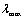) Trace Test (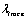) Test Statistics 5% CV Test Statistics 5% CV 0.16114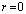44.2781 36.41 93.7320 77.74 0.08114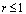21.3257 30.33 49.4539* 54.64 0.06097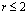15.8518 23.78 28.1282 34.55 0.04602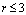11.8730 16.87 12.2764 18.17 0.00160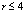0.4034 3.74 0.4034 3.74

To better analyse the dynamics of the macroeconomic variables we use the  two step methodology. From Table 6 below, the results on the inference column indicate that interest rate Granger causes real economic activity, which indicate that historical interest rates can be used to predict future real economic activities. However, past real economic activities cannot be used to predict future interest rates. Table 6 further shows that, inflation and real economic activity have among them a unidirectional causality. Specifically, inflation Granger causes real economic activities but not the reverse. The result further shows that there is bidirectional causality between interest rate and inflation. Thus, the historical values of each variable can be used to predict the other. The result on the inference on table 7 also shows evidence of no Granger causality between real economic activity and exchange rate, money supply respectively. There is some evidence of Granger causality between exchange rate and interest rate, money supply and interest rate.

Table 7. Summary results of the Granger causality test.

 Variables Direction of Causality χ2 Prob. Value Inference lnreal (Y); lnintr (X) X→Y 9.045** 0.021 Reject lnintr (Y); lnreal (X) X→Y 6.367 0.832 Do not reject lnreal (Y); lninf (X) X→Y 7.5733** 0.024 Reject lninf (Y); lnreal (X) X→Y 0.47923 0.787 Do not reject lnintr (Y); lninf (X) X→Y 15.445*** 0.000 Reject lninf (Y); lnintr (X) X→Y 14.1624*** 0.001 Reject lnreal (Y); lnexrate (X) X→Y 1.7637 0.414 Do not reject lnexrate (Y); lnreal (X) X→Y 3.8029 0.149 Do not reject lnreal (Y); lnm2 (X) X→Y 2.3872 0.303 Do not reject lnm2 (Y); lnreal (X) X→Y 4.3728 0.112 Do not reject lnexrate (Y); lnintr (X) X→Y 2.1036 0.349 Do not reject lnintr (Y); lnexrate (X) X→Y 7.628** 0.022 Reject lnm2 (Y); lninf (X) X→Y 1.6625 0.435 Do not reject lninf (Y); lnm2 (X) X→Y 0.48163 0.786 Do not reject lnintr (Y); lnm2 (X) X→Y 5.9325* 0.051 Reject lninf (Y); lnexrate (X) X→Y 2.5827 0.275 Do not reject lnm2 (Y); lnintr(X) X→Y 16.377*** 0.000 Reject Note: Null hypothesis: X¿Y (X does not Granger Cause Y); ***, **, * denotes rejection of null hypothesis at 1%, 5% and 10% significance levels respectively. → implies direction of causality.Fig. 2. Orthogonalised Impulse Response Functions.

4.3. Impulse Response Functions

The impulse response function of VAR is to analyze the dynamic effects of the system when the model received the impulse. We plot the orthogonal impulse responses from real economic activity following shocks from interest rates, inflation, exchange rate and oil prices. The plots are illustrated in Figure 2 and in each of the figure, the first variable is the impulse and the second variable is the response.

The figure 2 indicates that when the impulse is inflation, then every response of real sector activity is all positive at each responsive time period, but fluctuates. After the first month, following the shock, real activity responds positively and then after falls and remains positively until all the shocks die out. The response to the shocks from inflation is not stable as they wander about for the first month before it finally settles. Unsurprisingly, impulses from oil prices affects real economic activities negatively a month following the shock after which the response turns positive and dies out by the third month. Impulses from exchange rates also seem to have a similar pattern as shocks die out after few months. Responses from interest rates impulses are mild and hence remain quite low and stable until all the shocks are accommodated. Exchange rate shocks are contemporaneous deleterious to real economic activities after which the reaction becomes positive and subsequently dies away gradually. Real economic activity responds to shocks from exchange rate in the similar manner as that of inflation. Shocks die away by the fourth month as the reactions hover in a dampening manner.

5. Conclusion

The results show that there exists a unique long-run relationship between real economic activity and the other variables. Accordingly, inflation is identified as important determinant of real economic activity growth in Ghana. Conversely, real economic activity was found not to significantly determine inflation and interest rate within the period under investigation. The relationship between inflation and interest rate is, however, significant determined positive in either direction. This implies that the connection between inflation, interest rate and economic activity is not immediately automatic.

References

1. Mallik, G., & Chowdhury, A. (2001). Inflation and economic growth: evidence from four south Asian countries. Asia-Pacific Development Journal, 8(1), 123-135.
2. Albu, L. L. (2006). Trends in the interest rate-Investment-GDP Growth Relationship. Journal for Economic Forecasting, 3(3), 5-13.
3. Obamuyi, T. M. (2009). An investigation of the relationship between interest rates and economic growth in Nigeria, 1970-2006. Journal of economics and International Finance, 1(4), 093-098.
4. Etornam, D. K., & Denis, D. (2015). Granger Causality Analysis on Ghana’s Macro-Economic Performance and Oil Price Fluctuations. Journal of Resources Development and Management, 6, 1-5.
5. Al-Majali, A. A., & Al-Assaf, G. I. (2014). Long-run and short-run relationship between stock market index and main macroeconomic variables performance in Jordan. European Scientific Journal, 10(10).
6. McKinnon, R. I. (1973). Money and capital in economic development. Brookings Institution Press.
7. Shaw, 1973; ES Shaw; Financial deepening in economic development. Oxford University Press, New York, NY.
8. Engle, R. F., & Granger, C. W. (1987). Co-integration and error correction: representation, estimation, and testing. Econometrica: journal of the Econometric Society, 251-276.
9. Dickey, D. A., & Fuller, W. A. (1979). Distribution of the estimators for autoregressive time series with a unit root. Journal of the American statistical association, 74(366a), 427-431.
10. Phillips, P. C., & Perron, P. (1988). Testing for a unit root in time series regression. Biometrika, 75(2), 335-346.
11. MacKinnon, J. G., & Queen's University (Kingston, Ont.). Institute for Economic Research. (1995). Numerical distribution functions for unit root and cointegration tests. Institute for Economic Research, Queen's University.
12. Johansen, S. (1995). Identifying restrictions of linear equations with applications to simultaneous equations and cointegration. Journal of econometrics, 69(1), 111-132.
13. Johansen, S. (1988). Statistical analysis of cointegration vectors. Journal of economic dynamics and control, 12(2), 231-254.
14. Charemza, W. W., & Deadman, D. F. (1997). New Directions in Econometric Practice: General to Specific Modelling, Cointegration and Vector Autoregresion. Edward Elgar.
15. Granger, C. W. J., (1988): "Some Recent Developments in a Concept of Causality," Journal of Econometrics. Vol. 39, pp. 199-211.
16. Granger, C. W. J., (1969): "Investigating Causal Relations by Econometric Models and Cross Spectral Methods," Econometrica. Vol. 37, pp. 424-438.

Footnotes

 Cedi (¢) is Ghana’s official unit of currency generally denoted as GH¢. On 3rd July 2007, the Ghanaian cedi (GHC) was redenominated. The new Ghana cedi (GHS) is equal to 10,000 old Ghanaian Cedis (1 GHS = 10,000 GHC). The old currency remained in circulation alongside the new until December 2007. One Ghana cedi is divided into one hundred Ghana pesewas (Gp).

 Ghana, prior to drilling and exporting oil in commercial quantities was a net importer of crude oil, it had no influence on the oil price. Thus, using oil price as an exogenous factor is an innocuous assumption.

Table 4 indicates the VAR lag order selection criteria and the optimal lag chosen in this paper is 2 based on the AIC.

 Contents 1. 2. 3. 3.1. 3.2. 3.3. 4. 4.1. 4.2. 4.3. 5.
Article ToolsAbstractPDF(416K)